Mathematics: CUET Mock Test - 4

# Mathematics: CUET Mock Test - 4

Test Description

## 40 Questions MCQ Test CUET Science Subjects Mock Tests | Mathematics: CUET Mock Test - 4

Mathematics: CUET Mock Test - 4 for Class 12 2023 is part of CUET Science Subjects Mock Tests preparation. The Mathematics: CUET Mock Test - 4 questions and answers have been prepared according to the Class 12 exam syllabus.The Mathematics: CUET Mock Test - 4 MCQs are made for Class 12 2023 Exam. Find important definitions, questions, notes, meanings, examples, exercises, MCQs and online tests for Mathematics: CUET Mock Test - 4 below.
Solutions of Mathematics: CUET Mock Test - 4 questions in English are available as part of our CUET Science Subjects Mock Tests for Class 12 & Mathematics: CUET Mock Test - 4 solutions in Hindi for CUET Science Subjects Mock Tests course. Download more important topics, notes, lectures and mock test series for Class 12 Exam by signing up for free. Attempt Mathematics: CUET Mock Test - 4 | 40 questions in 45 minutes | Mock test for Class 12 preparation | Free important questions MCQ to study CUET Science Subjects Mock Tests for Class 12 Exam | Download free PDF with solutions
 1 Crore+ students have signed up on EduRev. Have you?
Mathematics: CUET Mock Test - 4 - Question 1

### Which one of the following is correct if we differentiate the equation xy = aex + be-x two times?

Detailed Solution for Mathematics: CUET Mock Test - 4 - Question 1

We have xy = aex + be-x ……(1)
Differentiating (1) with respect to x, we get
x(dy/dx) + y = aex + be-x …..(2)
Differentiating (2) now, with respect to x, we get
x(d2y/ dx) + dy/dx + dy/dx = aex + be-x
From (1),
aex + be-x = xy, so that we get
x(d2y/ dx) + 2(dy/dx) = xy
which is the required differential equation.

Mathematics: CUET Mock Test - 4 - Question 2

### What is the differential equation of all parabolas whose directrices are parallel to the x-axis?

Detailed Solution for Mathematics: CUET Mock Test - 4 - Question 2

The equation of family of parabolas is Ax2 + Bx + C = 0 where, A, B, C are arbitrary constant.
By differentiating the equation with respect to x till all the constants get eliminated,
Hence, d3y/dx3 = 0

Mathematics: CUET Mock Test - 4 - Question 3

### What will be the required solution of d2y/dx2 – 3dy/dx + 4y = 0?

Detailed Solution for Mathematics: CUET Mock Test - 4 - Question 3

d2y/dx2 – 3dy/dx + 4y = 0 …..(1)
Let, y = emx be a trial solution of (1), then,
⇒ dy/dx = memx and d2y/dx2 = m2emx
Clearly, y = emx will satisfy equation (1). Hence, we have,
m2emx – 3m * emx – 4emx = 0
⇒ m2 – 3m – 4 = 0 (as emx ≠ 0) …….(2)
⇒ m2 – 4m + m – 4 = 0
⇒ m(m – 4) + 1(m – 4) = 0
Or, (m – 4)(m + 1) = 0
Thus, m = 4 or m = -1
Clearly, the roots of the auxiliary equation (2) are real and unequal.
Therefore, the required general solution of (1) is
y = Ae4x + Be-x where A and B are constants.

Mathematics: CUET Mock Test - 4 - Question 4

What is the solution of the given equation (D + 1)2y = 0 given y = 2 loge 2 when x = loge 2 and y = (4/3) loge3 when x = loge3?

Detailed Solution for Mathematics: CUET Mock Test - 4 - Question 4

(D + 1)2y = 0
Or, (D2 + 2D+ 1)y = 0
⇒ d2y/dx2 + 2dy/dx + y = 0 ……….(1)
Let y = emx be a trial solution of equation (1). Then,
⇒ dy/dx = memx and d2y/dx2 = m2emx
Clearly, y = emx will satisfy equation (1). Hence, we have
⇒ m2.emx + 2m.emx + emx = 0
Or, m2 + 2m +1 = 0 (as, emx ≠ 0) ………..(2)
Or, (m + 1)2 = 0
⇒ m = -1, -1
So, the roots of the auxiliary equation (2) are real and equal. Therefore, the general solution of equation (1) is
y = (A + Bx)e-x where A and B are two independent arbitrary constants ……….(3)
Given, y = 2 loge 2 when x = loge 2
Therefore, from (3) we get,
2 loge 2 = (A + B loge2)e-x
Or, 1/2(A + B loge2) = 2 log e2
Or, A + B loge2 = 4 loge2 ……….(4)
Again y = (4/3) loge3 when x = loge3
So, from (3) we get,
4/3 loge3 = (A + Bloge3)
Or, A + Bloge3 = 4loge3 ……….(5)
Now, (5) – (4) gives,
B(loge3 – loge2) = 4(loge3 – loge2)
⇒ B = 4
Putting B = 4 in (4) we get, A = 0
Thus the required solution of (1) is y = 4xe-x

Mathematics: CUET Mock Test - 4 - Question 5

If, A and B are arbitrary constants then what will be the differential equation of y = Ax + B/x?

Detailed Solution for Mathematics: CUET Mock Test - 4 - Question 5

Given, y = Ax + B/x
⇒ xy = Ax2 + B ……….(1)
Differentiating (1) with respect to x, we get,
d(xy)/dx = d/dx(Ax2 + B)
or, xdy/dx + y = A * 2x ……….(2)
Differentiating again with respect to x, we get,
x*d2y/dx2 + dy/dx + dy/dx = A*2 ……….(3)
Eliminating A from (2) and (3) we get,
x2 d2 y/dx2 + 2xdy/dx = 2Ax  [multiplying (3) by x]
or, x2 d2 y/dx2 + 2xdy/dx = xdy/dx + y [using (2)]
or, x2 d2 y/dx2 + xdy/dx – y = 0

Mathematics: CUET Mock Test - 4 - Question 6

What will be the value of C if C the constant of the coefficient of the solution of the given equation (D + 1)2y = 0 given y = 2 loge 2 when x = loge 2 and y = (4/3) loge3 when x = loge3?

Detailed Solution for Mathematics: CUET Mock Test - 4 - Question 6

(D + 1)2y = 0
Or, (D2 + 2D+ 1)y = 0
⇒ d2y/dx2 + 2dy/dx + y = 0 ……….(1)
Let y = emx be a trial solution of equation (1). Then,
⇒ dy/dx = memx and d2y/dx2 = m2emx
Clearly, y = emx will satisfy equation (1). Hence, we have
⇒ m2.emx + 2m.emx + emx = 0
Or, m2 + 2m + 1 = 0 (as, emx ≠ 0) ………..(2)
Or, (m + 1)2 = 0
⇒ m = -1, -1
So, the roots of the auxiliary equation (2) are real and equal. Therefore, the general solution of equation (1) is
y = (A + Bx)e-x where A and B are two independent arbitrary constants ……….(3)
Given, y = 2 loge 2 when x = loge 2
Therefore, from (3) we get,
2 loge 2 = (A + B loge2)e-x
Or, 1/2(A + B loge2) = 2 log e2
Or, A + B loge2 = 4 loge2 ……….(4)
Again y = (4/3) loge3 when x = loge3
So, from (3) we get,
4/3 loge3 = (A + Bloge3)
Or, A + Bloge3 = 4loge3 ……….(5)
Now, (5) – (4) gives,
B(loge3 – loge2) = 4(loge3 – loge2)
⇒ B = 4
Putting B = 4 in (4) we get, A = 0
Thus the required solution of (1) is y = 4xe-x
So, C = 4

Mathematics: CUET Mock Test - 4 - Question 7

What is thedifferential equation whose solution represents the family y = ae3x + bex?

Detailed Solution for Mathematics: CUET Mock Test - 4 - Question 7

The original given equation is,
y = ae3x + bex ……….(1)
Differentiating the above equation, we get
dy/dx = 3ae3x + bex ……….(2)
d2y/dx2 = 9ae3x + bex ……….(3)
Now, to obtain the final equation we have to make the RHS = 0,
So, to get RHS = 0, we multiply, (1) with 3 and (2) with -4,
Thus , 3y = 3ae3x + 3bex ……….(4)
And -4(dy/dx) = -12ae3x – 4 bex ……….(5)
Now, adding the above three equations,(i.e. (3) + (4) + (5)) we get,
d2y/dx2 – 4dy/dx + 3y = 0

Mathematics: CUET Mock Test - 4 - Question 8

If y = t(x) be a differentiable function ᵾ x € R, then which of the following is always true?

Detailed Solution for Mathematics: CUET Mock Test - 4 - Question 8

(dy/dx)= (dx/dy)-1
So, d2y/dx2 = – (dx/dy)-2 d/dx(dx/dy)
= – (dy/dx)2(d2x/dy2)(dy/dx)
⇒ d2y/dx2 + (dy/dx)3 d2x/dy2 = 0

Mathematics: CUET Mock Test - 4 - Question 9

What will be the general solution of the differential equation d2y/dx2 = e2x(12 cos3x – 5 sin3x)? (here, A and B are integration constant)

Detailed Solution for Mathematics: CUET Mock Test - 4 - Question 9

Given, d2y/dx2 = e2x(12 cos3x – 5 sin3x) ………(1)
Integrating (1) we get,
dy/dx = 12∫ e2xcos3x dx – 5∫ e2x sin3x dx
= 12 * (e2x/22 + 32)[2cos3x + 3sin3x] – 5 * (e2x/22 + 32)[2sin3x – 3cos3x] + A (A is integrationconstant)
So dy/dx = e2x/13 [24 cos3x + 36 sin3x – 10 sin3x + 15 cos3x] + A
= e2x/13(39 cos3x + 26 sin3x) + A
⇒ dy/dx = e2x(3 cos3x + 2 sin3x) + A ……….(2)
Again integrating (2) we get,
y = 3*∫ e2x cos3xdx + 2∫ e2x sin3xdx + A ∫dx
y = 3*(e2x/22 + 32)[2cos3x + 3sin3x] + 2*(e2x/22 + 32)[2sin3x – 3cos3x] + Ax + B (B is integration constant)
y = e2x/13(6 cos3x + 9 sin3x + 4 sin3x – 6 cos3x) + Ax + B
or, y = e2x sin3x + Ax + B

Mathematics: CUET Mock Test - 4 - Question 10

Which of the following is the valid differential equation x = a cos(αt + β)?

Detailed Solution for Mathematics: CUET Mock Test - 4 - Question 10

Since, x = a cos(αt + β)
Therefore, dx/dt = a cos(αt + β)
And, d2x/dt2 = -a α2 cos(αt + β)
= -α2 a cos(αt + β)
Or, d2x/dt2 = -α2 [as a cos(αt + β) = x]
So, d2x/dt2 + α2x = 0

Mathematics: CUET Mock Test - 4 - Question 11

If, A normal is drawn at a point P(x, y) of a curve. It meets the x-axis at Q. If PQ is of constant length k. What kind of curve is passing through (0, k)?

Detailed Solution for Mathematics: CUET Mock Test - 4 - Question 11

Equation of the normal at a point P(x, y) is given by
Y – y = -1/(dy/dx)(X – x) ….(1)
Let the point Q at the x-axis be (x1 , 0).
From (1), we get
y(dy/dx) = x1 – x ….(2)
Now, giving that PQ2 = k2
Or, x1 – x + y2 = k2
=>y(dy/dx) = ± √(k2 – y2) ….(3)
(3) is the required differential equation for such curves,
Now solving (3) we get,
∫-dy/√(k2 – y2) = ∫-dx
Or, x2 + y2 = k2 passes through (0, k)
Thus, it is a circle.

Mathematics: CUET Mock Test - 4 - Question 12

What is the solution of (y(dy/dx) + 2x)2 = (y2 + 2x2)[1 + (dy/dx)2]?

Detailed Solution for Mathematics: CUET Mock Test - 4 - Question 12

Here, y2(dy/dx)2 + 4x2 + 4xy(dy/dx) = (y2 + 2x2)[1 + (dy/dx)2]
⇒ dy/dx = y/x ± √(1/2(y/x)2) + 1
Let, y = vx
⇒ v + x dv/dx = v ± √(1/2(v)2) + 1
Integrating both sides,
±√dv/(√(1/2(v)2) + 1) = ∫dx/x
cx±1/√2 = y/x + √(y2 + 2x2)/x2

Mathematics: CUET Mock Test - 4 - Question 13

A particle starts from the origin with a velocity 5cm/sec and moves in a straight line, its acceleration at time t seconds being (3t2 – 5t)cm/sec2. What will be the velocity of the particle?

Detailed Solution for Mathematics: CUET Mock Test - 4 - Question 13

Let x cm be the distance of the moving particle from the origin and v cm/sec be its velocity at the end of t seconds. Then the acceleration of the particle at time t seconds = dv/dt and its velocity at that time = v = dx/dt.
By question, dv/dt = 3t2 – 5t
Or, dv = 3t2 dt – 5tdt
Or, ∫dv = 3∫t2 dt – 5∫t dt
Or, v = t3 – (5/2)t2 + c ……….(1)
Given, v = 5, when t = 0; hence putting these values in equation (1) we get, c = 5
Thus v = t3 – (5/2)t2 + 5
Or, dx/dt = t3 – (5/2)t2 + 5 ………..(2)
Thus, the velocity of the particle at the end of 4 seconds,
= [v]t = 4 = (43 – (5/2)42 + 5 ) cm/sec [putting t = 4 in (2)]
= 29 cm/sec

Mathematics: CUET Mock Test - 4 - Question 14

What will be the differential equation form of √(a2 + x2)dy/dx + y = √(a2 + x2) – x?

Detailed Solution for Mathematics: CUET Mock Test - 4 - Question 14

The given form of equation can be written as,
dy/dx + 1/√(a2 + x2) * y = (√(a2 + x2) – x)/√(a2 + x2) ……(1)
We have, ∫1/√(a2 + x2)dx = log(x + √(a2 + x2))
Therefore, integrating factor is,
e∫1/√(a2 + x2) = elog(x + √(a2 + x2))
= x + √(a2 + x2)
Therefore, multiplying both sides of (1) by x + √(a2 + x2) we get,
x + √(a2 + x2dy/dx + (x + √(a2 + x2))/ √(a2 + x2)*y = (x + √(a2 + x2))(√(a2 + x2) – x)/√(a2 + x2)
or, d/dx[x + √(a2 + x2)*y] = (a2 + x2) ………..(2)
Integrating both sides of (2) we get,
(x + √(a2 + x2) * y = a2∫dx/√(a2 + x2)
= a2 log (x + √(a2 + x2)) + c

Mathematics: CUET Mock Test - 4 - Question 15

A curve passes through (1, 1) such that the triangle formed by the coordinate axes and the tangent at any point of the curve is in the first quadrant and has its area equal to 2. What will be the equation of the curve?

Detailed Solution for Mathematics: CUET Mock Test - 4 - Question 15

The equation of tangent to the curve y = f(x), at point (x, y), is
Y – y = dy/dx * (X – x) …..(1)
Where it meets the x axis, Y = 0 and X = (x – y/(dy/dx))
Where it meets the y axis, X = 0 and Y = (y – x/(dy/dx))
Also, the area of the triangle formed by (1) with the coordinate axes is 2, so that,
(x – y/(dy/dx))* (y – x/(dy/dx)) = 4
Or, (y – x/(dy/dx))2 – 4dy/dx = 0
Or, x2(dy/dx)2 – 2(xy – 2)dy/dx + y2 = 0
Solving for dy/dx we get,
dy/dx = [(xy – 2) ± √(1 – xy)]/ x2
Let, 1 – xy = t2
⇒ x(dy/dx) + y = -2t(dt/dx)
⇒ x2(dy/dx) = t2 – 1 – 2tx(dt/dx), so that (3) gives
t(x(dt/dx) – (t ± 1)) = 0
Hence, either t = 0
⇒ xy = 1 which is satisfied by (1, 1)
Or, x dt/dx = t ± 1
⇒ dx/x = dt/t ± 1
⇒ t ± 1 = cx
For x = 1, y = 1 and t = 0
⇒ c = ± 1, so the solution is
t = ± (x – 1) => t2 = (x – 1)2
Or, 1 – xy = x2 – 2x + 1
Or, x + y = 2
Thus, the two curves that satisfies are xy = 1 and x + y = 2

Mathematics: CUET Mock Test - 4 - Question 16

A curve passes through (1, 1) such that the triangle formed by the coordinate axes and the tangent at any point of the curve is in the first quadrant and has its area equal to 2. What is the differential equation?

Detailed Solution for Mathematics: CUET Mock Test - 4 - Question 16

The equation of tangent to the curve y = f(x), at point (x, y), is
Y – y = dy/dx * (X – x) …..(1)
Where it meets the x axis, Y = 0 and X = (x – y/(dy/dx))
Where it meets the y axis, X = 0 and Y = (y – x/(dy/dx))
Also, the area of the triangle formed by (1) with the coordinate axes is 2, so that,
(x – y/(dy/dx))* (y – x/(dy/dx)) = 4
Or, (y – x/(dy/dx))2 – 4dy/dx = 0
Or, x2(dy/dx)2 – 2(xy – 2)dy/dx + y2 = 0
Solving for dy/dx we get,
dy/dx = [(xy – 2) ± √(1 – xy)]/ x2

Mathematics: CUET Mock Test - 4 - Question 17

What will be the value of dy/dx – a/x * y = (x + 1)/x?

Detailed Solution for Mathematics: CUET Mock Test - 4 - Question 17

dy/dx – a/x * y = (x + 1)/x …….(1)
Multiplying both sides of equation (1) by
e∫-a/xdx
= e-a log x
= elog x-a
= x-a
We get, x-ady/dx – x-a (a/x)y = x-a (x + 1)/x
Or, d/dx(y . x-a) = x-a + x-a – 1 …….(2)
Integrating both sides of (2) we get,
y. x-a = x-a + 1/(-a + 1) + x-a – 1 + 1/(-a -1 + 1) + c
= x-a.x/(1 – a) + x-a/-a + c
Or, y = x/(1 – a) – 1/a + cxa

Mathematics: CUET Mock Test - 4 - Question 18

What is the solution of dy/dx = (6x + 9y – 7)/(2x + 3y – 6)?

Detailed Solution for Mathematics: CUET Mock Test - 4 - Question 18

dy/dx = (6x + 9y – 7)/(2x + 3y – 6)
So, dy/dx = (3(2x + 3y) – 7)/(2x + 3x – 6) ……….(1)
Now, we put, 2x + 3y = z
Therefore, 2 + 3dy/dx = dz/dx [differentiating with respect to x]
Or, dy/dx = 1/3(dz/dx – 2)
Therefore, from (1) we get,
1/3(dz/dx – 2) = (3z – 7)/(z – 6)
Or, dz/dx = 2 + (3(3z – 7))/(z – 6)
= 11(z – 3)/(z – 6)
Or, (z – 6)/(z – 3) dz = 11 dx
Or, ∫(z – 6)/(z – 3) dz = ∫11 dx
Or, ∫(1 – 3/(z – 3)) dz = 11x + c
Or, z – log |z – 3| = 11x + c
Or, 2x + 3y – 11x – 3log|2x + 3y -3| = c
Or, 3y – 9x – 3log|2x + 3y – 3| = c
Or, 3x – y + log|2x + 3y – 3| = -c/3

Mathematics: CUET Mock Test - 4 - Question 19

A particle starts from the origin with a velocity 5cm/sec and moves in a straight line, its acceleration at time t seconds being (3t2 – 5t)cm/sec2. What will be the distance from the origin at the end of 4 seconds?

Detailed Solution for Mathematics: CUET Mock Test - 4 - Question 19

Let x cm be the distance of the moving particle from the origin and v cm/sec be its velocity at the end of t seconds. Then the acceleration of the particle at time t seconds = dv/dt and its velocity at that time = v = dx/dt.
By question, dv/dt = 3t2 – 5t
Or, dv = 3t2 dt – 5t dt
Or, ∫dv = 3∫t2 dt – 5∫t dt
Or, v = t3 – (5/2)t2 + c ……….(1)
Given, v = 5, when t = 0; hence putting these values in equation (1) we get, c = 5
Thus v = t3 – (5/2)t2 + 5
Or, dx/dt = t3 – (5/2)t2 + 5 ………..(2)
Or, dx = t3 dt – (5/2)t2 dt + 5 dt
Integrating this we get,
x = (1/4)t4 – (5/2)t3/3 + 5t + k ……….(3)
By the problem, x = 0, when t = 0; hence, from (3) we get, k = 0.
Thus, x = (1/4)t4 – (5/6)t3 + 5t ……….(4)
Thus, the velocity of the particle at the end of 4 seconds,
= [x]t = 4 = (1/4)44 – (5/6)43 + 5(4) [putting t = 4 in (4)]
= 30(2/3) cm

Mathematics: CUET Mock Test - 4 - Question 20

What is the equation of the curve passing through (1, 0) of (y(dy/dx) + 2x)2 = (y2 + 2x2)[1 + (dy/dx)2]?

Detailed Solution for Mathematics: CUET Mock Test - 4 - Question 20

Here, y2(dy/dx)2 + 4x2 + 4xy(dy/dx) = (y2 + 2x2)[1 + (dy/dx)2]
⇒ dy/dx = y/x ± √(1/2(y/x)2) + 1
Let, y = vx
⇒ v + x dv/dx = v ± √(1/2(v)2) + 1
Integrating both sides,
±∫dv/(√(1/2(v)2) + 1) = ∫dx/x
cx±1/√2 = y/x + √(y2 + 2x2)/x2 (put v/√2 = tan t)
putting x = 1, y = 0, we get c = √2
So, the curve is given by,
√2x±1/√2 = y/x + √(y2 + 2x2)/x2

Mathematics: CUET Mock Test - 4 - Question 21

Find the general solution of the differential equation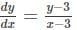(x, y≠3).

Detailed Solution for Mathematics: CUET Mock Test - 4 - Question 21

Given that,Separating the variables, we get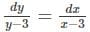log⁡(y - 3) = log⁡(x - 3) + log⁡C1
log⁡(y - 3) - log⁡(x - 3) = log⁡C1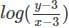= log⁡C1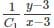= 0
y - 3 = 0 is the general solution for the given differential equation.

Mathematics: CUET Mock Test - 4 - Question 22

Find the general solution of the differential equation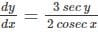.

Detailed Solution for Mathematics: CUET Mock Test - 4 - Question 22

Given that,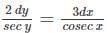Separating the variables, we get
2 cos⁡y dy = 3 sin⁡x dx
Integrating both sides, we get
∫ 2 cos⁡y dy = ∫ 3 sin⁡x dx
2 sin⁡y = 3(-cos⁡x) + C
3 cos⁡x + 2 sin⁡y = C

Mathematics: CUET Mock Test - 4 - Question 23

Find the general solution of the differential equation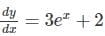Detailed Solution for Mathematics: CUET Mock Test - 4 - Question 23

Given that,Separating the variables, we get
dy = (3e+ 2)dx
Integrating both sides, we get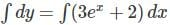-----(1)
y = 3e+ 2x + C which is the general solution of the given differential equation.

Mathematics: CUET Mock Test - 4 - Question 24

Find the particular solution of the differential equation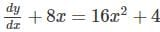given that y = 1/3 when x = 1.

Detailed Solution for Mathematics: CUET Mock Test - 4 - Question 24

Given that,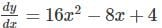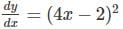Separating the variables, we get
dy = (4x - 2)2 dx
Integrating both sides, we get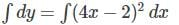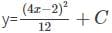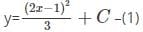Given that, y = 1/3 when x = 1
Therefore, equation (1) becomes,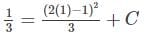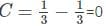Hence, the particular solution for the given differential solution is y =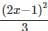Mathematics: CUET Mock Test - 4 - Question 25

Find the particular solution of the differential equation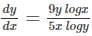.

Detailed Solution for Mathematics: CUET Mock Test - 4 - Question 25Separating the variables, we get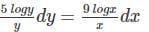Integrating both sides, we get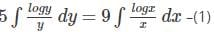First, for integrating logy/y
Let log⁡y = t
Differentiating w.r.t y, we get
1/y dy=dt
∴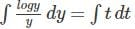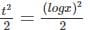Hence, equation (1), becomes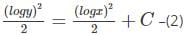Given y = 2, we get x = 2
Substituting the values in equation (2), we get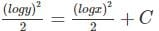C = 0
Therefore, the equation becomes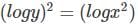∴ (log⁡y)2  - (log⁡x)2 = 0.

Mathematics: CUET Mock Test - 4 - Question 26

Find the particular solution for the differential equation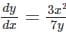given that, y = 1 when x = 1.

Detailed Solution for Mathematics: CUET Mock Test - 4 - Question 26

Given that,Separating the variables, we get
7y dy = 3x2 dx
Integrating both sides, we get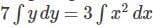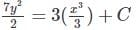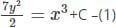Given that y = 1, when x = 1
Substituting the values in equation (1), we get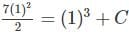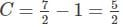Hence, the particular solution of the given differential equation is: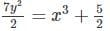⇒ 7y= 2x+ 5

Mathematics: CUET Mock Test - 4 - Question 27

Which of the following functions is the solution of the differential equation dydx + 2y = 0?

Detailed Solution for Mathematics: CUET Mock Test - 4 - Question 27

Consider the function y = e-2x
Differentiating both sides w.r.t x, we getdy/dx = -2y
⇒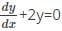Mathematics: CUET Mock Test - 4 - Question 28

Which of the following functions is a solution for the differential equation xy’ - y = 0?

Detailed Solution for Mathematics: CUET Mock Test - 4 - Question 28

Consider the function y = 2x
Differentiating w.r.t x, we get
y’= dy/dx = 2
Substituting in the equation xy’-y, we get
xy’- y = x(2) - 2x = 2x - 2x = 0
Therefore, the function y = 2x is a solution for the differential equation xy’ - y = 0.

Mathematics: CUET Mock Test - 4 - Question 29

Which of the following functions is a solution for the differential equation y” + 6y = 0?

Detailed Solution for Mathematics: CUET Mock Test - 4 - Question 29

Consider the function y = 5 cos⁡3x
Differentiating w.r.t x, we get
y’ = dy/dx = -15 sin⁡3x
Differentiating again w.r.t x, we get
y” = d2y/dx2 = -30 cos⁡3x
⇒ y” + 6y=0.
Hence, the function y = 5 cos⁡3x is a solution for the differential equation y” + 6y = 0.

Mathematics: CUET Mock Test - 4 - Question 30

Which of the following differential equations given below has the solution y = log⁡x?

Detailed Solution for Mathematics: CUET Mock Test - 4 - Question 30

Consider the function y = log⁡x
Differentiating w.r.t x, we get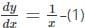Differentiating (1) w.r.t x, we get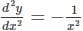∴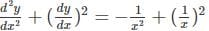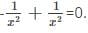Mathematics: CUET Mock Test - 4 - Question 31

The number of arbitrary constants in a particular solution of a fourth order differential equation is ______

Detailed Solution for Mathematics: CUET Mock Test - 4 - Question 31

The number of arbitrary constants for a particular solution of nth order differential equation is always zero.

Mathematics: CUET Mock Test - 4 - Question 32

Which of the following is not a possible ordered pair for a matrix with 6 elements.

Detailed Solution for Mathematics: CUET Mock Test - 4 - Question 32

The possible orders in which the matrix with 6 elements can be formed are 2×3, 3×2, 1×6, 6×1. Therefore, the possible orders pairs are (2,3), (3,2), (1,6), (6,1). Thus, (3,1) is not possible.

Mathematics: CUET Mock Test - 4 - Question 33

How many arbitrary constants will be there in the general solution of a second order differential equation?

Detailed Solution for Mathematics: CUET Mock Test - 4 - Question 33

The number of arbitrary constants in a general solution of a nth order differential equation is n.
Therefore, the number of arbitrary constants in the general solution of a second order D.E is 2.

Mathematics: CUET Mock Test - 4 - Question 34

Which of the following functions is a solution for the differential equation dy/dx -14x = 0?

Detailed Solution for Mathematics: CUET Mock Test - 4 - Question 34

Consider the function y = 7x2
Differentiating w.r.t x, we get
dy/dx -14x
⇒ dy/dx -14x = 0
Hence, the function y = 7x2 is a solution for the differential equation dydx - 14x = 0

Mathematics: CUET Mock Test - 4 - Question 35

Which of the following differential equations has the solution y = 3x2?

Detailed Solution for Mathematics: CUET Mock Test - 4 - Question 35

Consider the function y = 3x2
Differentiating w.r.t x, we get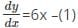Differentiating (1) w.r.t x, we get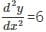∴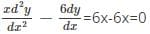Hence, the function y = 3x2 is a solution for the differential equation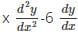= 0

Mathematics: CUET Mock Test - 4 - Question 36

What is the order of the matrix A=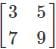?

Detailed Solution for Mathematics: CUET Mock Test - 4 - Question 36

The number of rows (m) and the number of columns (n) in the given matrix A=is 2. Therefore, the order of the matrix is 2×2(m×n)

Mathematics: CUET Mock Test - 4 - Question 37

What is the difference property of definite integrals?

Detailed Solution for Mathematics: CUET Mock Test - 4 - Question 37

The sum difference property of definite integrals is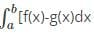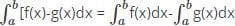Mathematics: CUET Mock Test - 4 - Question 38

What is the constant multiple property of definite integrals?

Detailed Solution for Mathematics: CUET Mock Test - 4 - Question 38

The constant multiple property of definite integrals is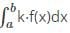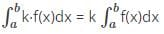Mathematics: CUET Mock Test - 4 - Question 39

Identify the zero-length interval property.

Detailed Solution for Mathematics: CUET Mock Test - 4 - Question 39

The zero-length interval property is one of the properties used in definite integrals and they are always positive. The zero-length interval property is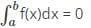.

Mathematics: CUET Mock Test - 4 - Question 40

What is the name of the property of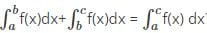?

Detailed Solution for Mathematics: CUET Mock Test - 4 - Question 40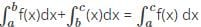is a property of definite integrals.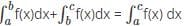is called as adding intervals property used to combine a lower limit and upper limit of two different integrals.

## CUET Science Subjects Mock Tests

60 tests
 Use Code STAYHOME200 and get INR 200 additional OFF Use Coupon Code
Information about Mathematics: CUET Mock Test - 4 Page
In this test you can find the Exam questions for Mathematics: CUET Mock Test - 4 solved & explained in the simplest way possible. Besides giving Questions and answers for Mathematics: CUET Mock Test - 4, EduRev gives you an ample number of Online tests for practice

60 tests